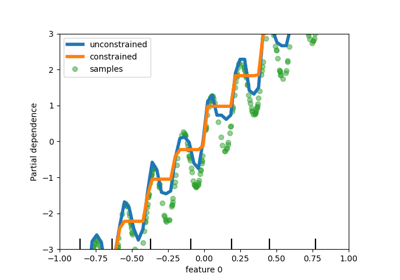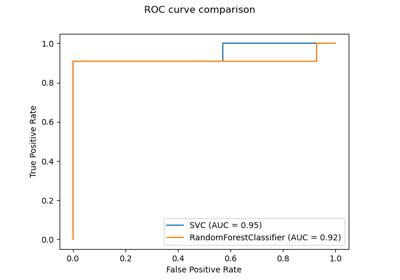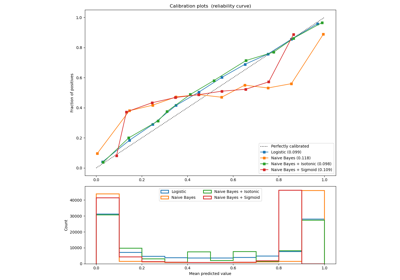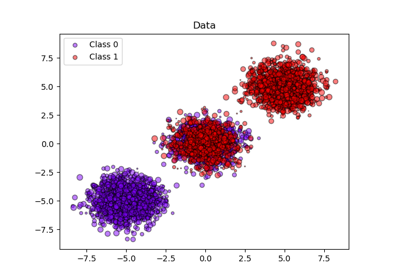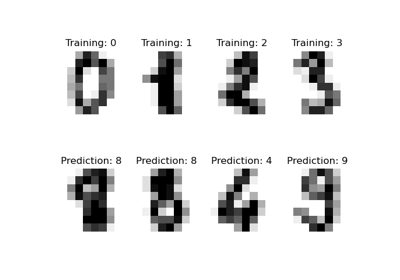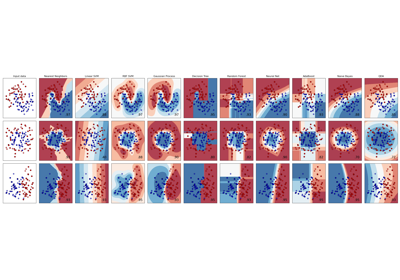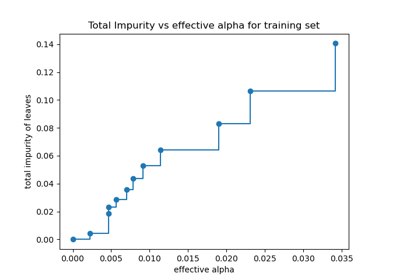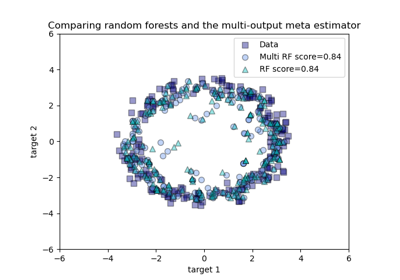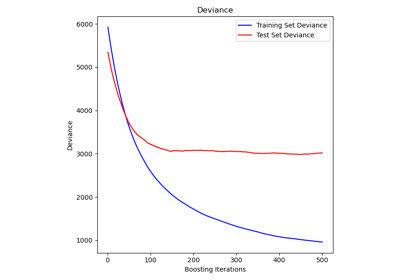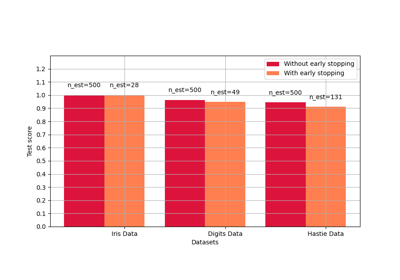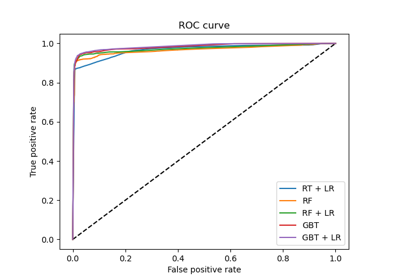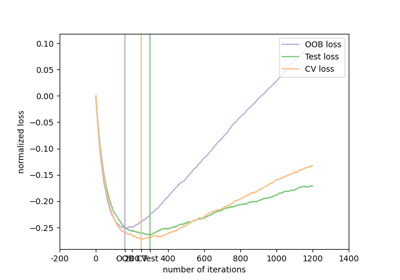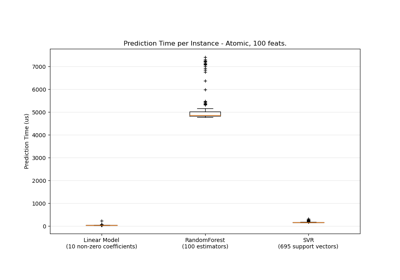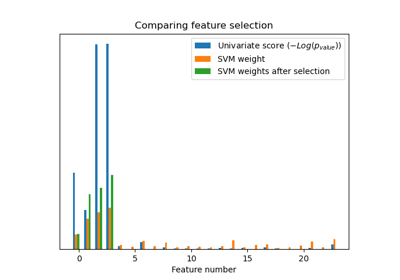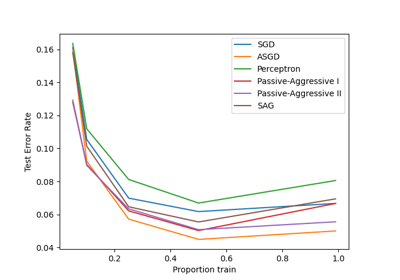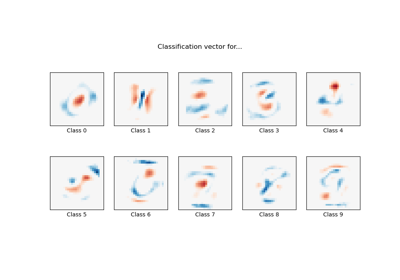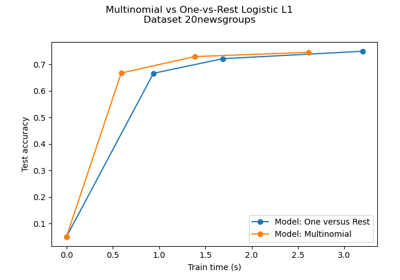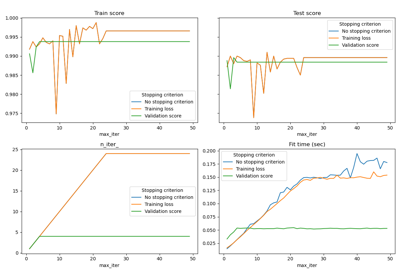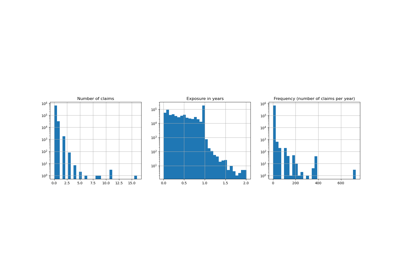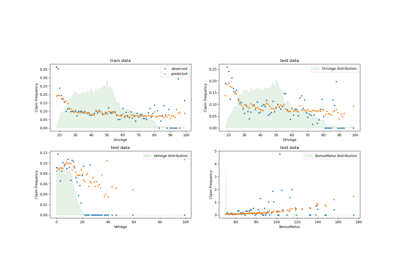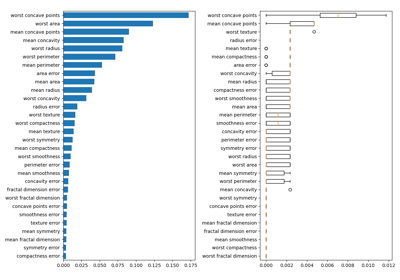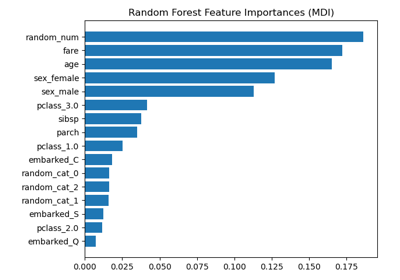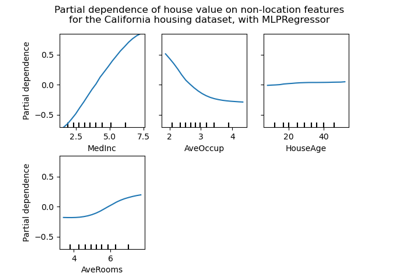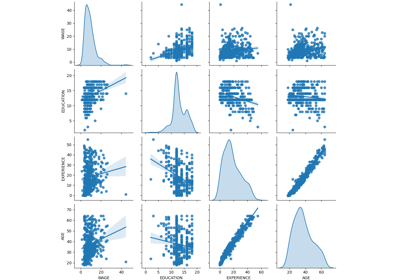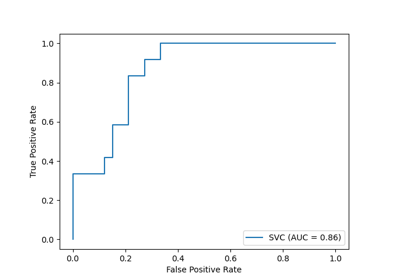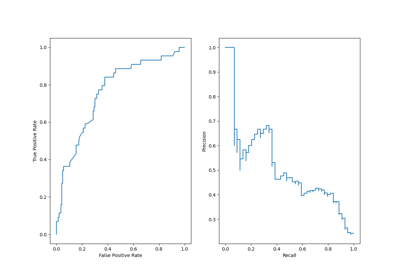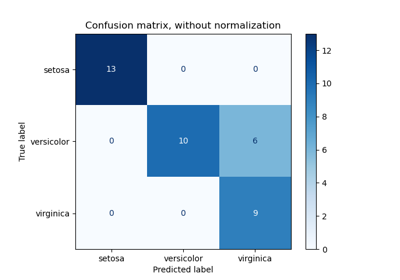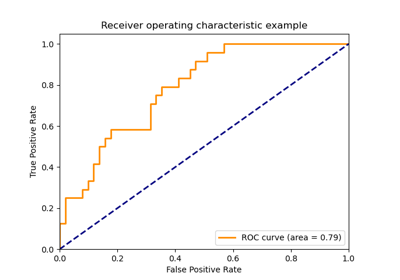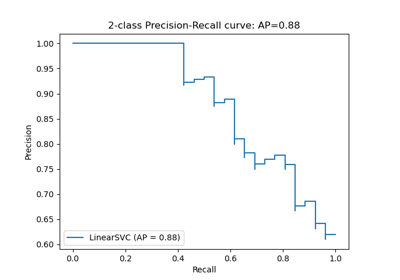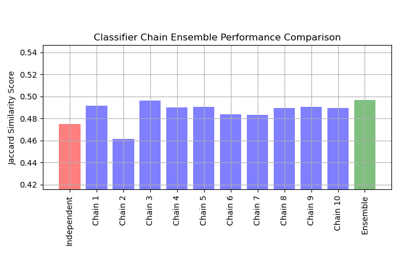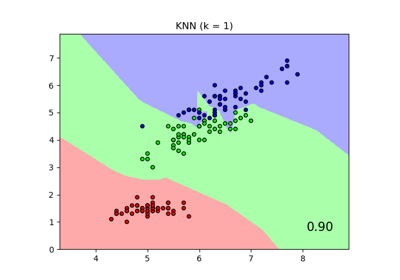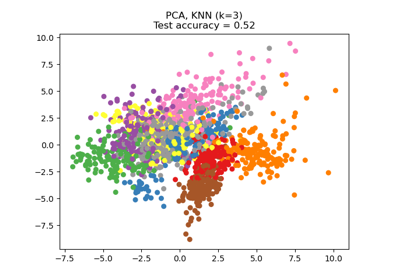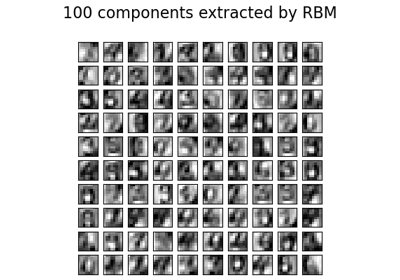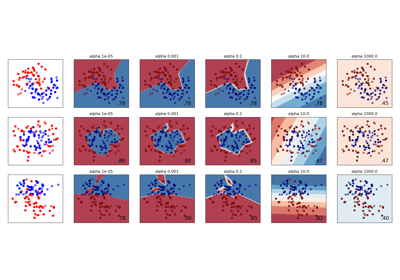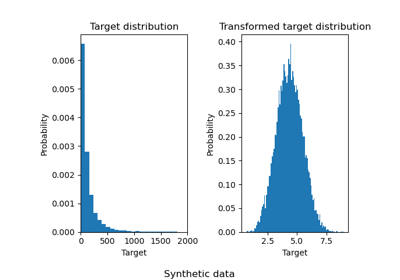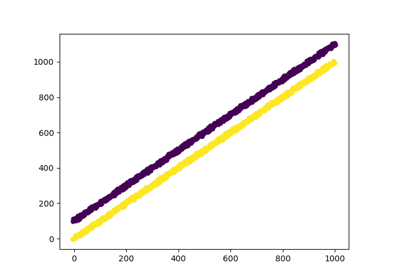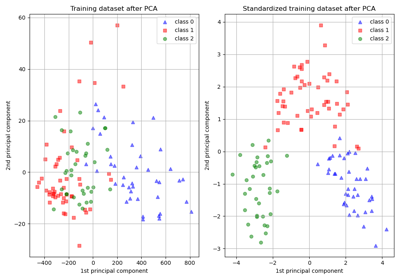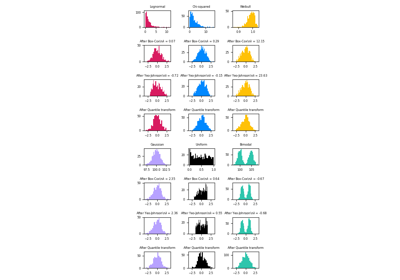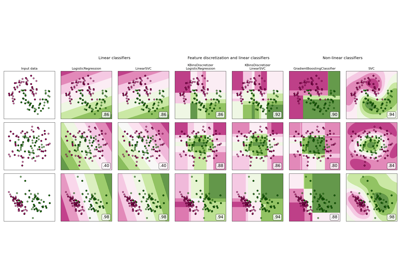# sklearn.model_selection.train_test_split¶

sklearn.model_selection.train_test_split(*arrays, **options)[source]

Split arrays or matrices into random train and test subsets

Quick utility that wraps input validation and next(ShuffleSplit().split(X, y)) and application to input data into a single call for splitting (and optionally subsampling) data in a oneliner.

Read more in the User Guide.

Parameters
*arrayssequence of indexables with same length / shape

Allowed inputs are lists, numpy arrays, scipy-sparse matrices or pandas dataframes.

test_sizefloat or int, default=None

If float, should be between 0.0 and 1.0 and represent the proportion of the dataset to include in the test split. If int, represents the absolute number of test samples. If None, the value is set to the complement of the train size. If train_size is also None, it will be set to 0.25.

train_sizefloat or int, default=None

If float, should be between 0.0 and 1.0 and represent the proportion of the dataset to include in the train split. If int, represents the absolute number of train samples. If None, the value is automatically set to the complement of the test size.

random_stateint or RandomState instance, default=None

Controls the shuffling applied to the data before applying the split. Pass an int for reproducible output across multiple function calls. See Glossary.

shufflebool, default=True

Whether or not to shuffle the data before splitting. If shuffle=False then stratify must be None.

stratifyarray-like, default=None

If not None, data is split in a stratified fashion, using this as the class labels.

Returns
splittinglist, length=2 * len(arrays)

List containing train-test split of inputs.

New in version 0.16: If the input is sparse, the output will be a scipy.sparse.csr_matrix. Else, output type is the same as the input type.

Examples

>>> import numpy as np
>>> from sklearn.model_selection import train_test_split
>>> X, y = np.arange(10).reshape((5, 2)), range(5)
>>> X
array([[0, 1],
[2, 3],
[4, 5],
[6, 7],
[8, 9]])
>>> list(y)
[0, 1, 2, 3, 4]

>>> X_train, X_test, y_train, y_test = train_test_split(
...     X, y, test_size=0.33, random_state=42)
...
>>> X_train
array([[4, 5],
[0, 1],
[6, 7]])
>>> y_train
[2, 0, 3]
>>> X_test
array([[2, 3],
[8, 9]])
>>> y_test
[1, 4]

>>> train_test_split(y, shuffle=False)
[[0, 1, 2], [3, 4]]


## Examples using sklearn.model_selection.train_test_split¶